##### Basic Math & Pre-Algebra For Dummies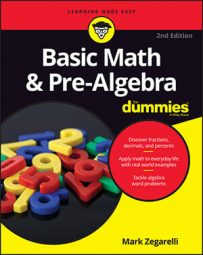The percent circle is a simple visual aid that helps you make sense of percent problems so that you can solve them easily. The trick to using a percent circle is to write information into it. For example, this image shows how to record the information that 50% of 2 is 1. In the percent circle, the ending number is at the top, the percentage is at the left, and the starting number is at the right.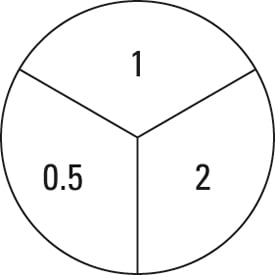Notice that, as you fill in the percent circle, you change the percentage, 50%, to its decimal equivalent, 0.5.

Here are the two main features of the percent circle:

• When you multiply the two bottom numbers together, they equal the top number:

0.5 × 2 = 1
• If you make a fraction out of the top number and either bottom number, that fraction equals the other bottom number: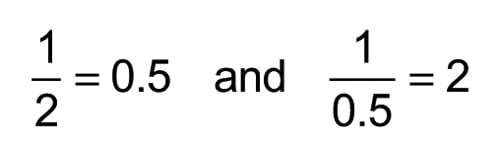These features are the heart and soul of the percent circle. They enable you to solve any of the three types of percent problems quickly and easily.

Most percent problems give you enough information to fill in two of the three sections of the percent circle. But no matter which two sections you fill in, you can find the number in the third section.

## Finding the ending number

Suppose you want to find out the answer to this problem:

What is 75% of 20?

You're given the percent and the starting number, and you're asked to find the ending number. To use the percent circle on this problem, fill in the information: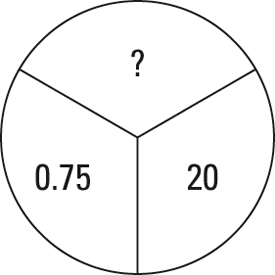Because 0.75 and 20 are both bottom numbers in the circle, multiply them to get the answer: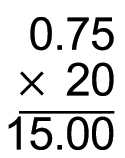So 75% of 20 is 15.

## Discovering the percentage

In the second type of problem, you get both the starting and ending numbers and have to find the percentage. Here's an example:

What percent of 50 is 35?

In this case, the starting number is 50 and the ending number is 35. Set up the problem on the percent circle as shown in the figure below.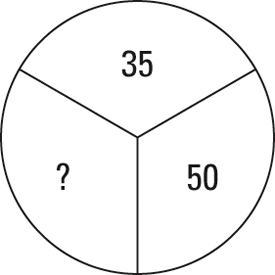This time, 35 is above 50, so make a fraction out of these two numbers: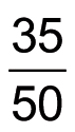This fraction is your answer, and all you have to do is convert the fraction to a percent. First, convert the fraction to a decimal: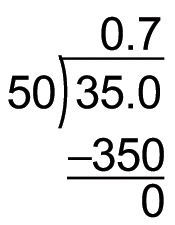Now convert 0.7 to a percent:

0.7 = 70%

## Tracking down the starting number

In the third type of problem, you get the percentage and the ending number, and you have to find the starting number. For example,

15% of what number is 18?

This time, the percentage is 15 and the ending number is 18, so fill in the percent circle as shown in the following figure.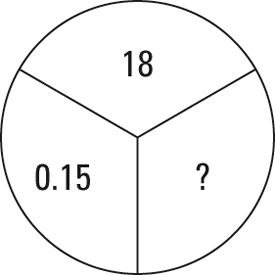Because 18 is above 0.15 in the circle, make a fraction out of these two numbers: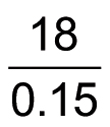This fraction is your answer; you just need to convert to a decimal: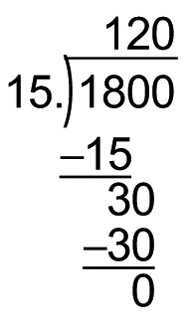In this case, the "decimal" you find is the whole number 120, so 15% of 120 is 18.